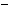Labware - MA35 Multivariable Calculus - Polar Coordinate Labs
 MA35 Labs » Polar Coordinate Labs Contents.1 Functions of Two Variables .1.1 Introduction .1.3 Slice Curves .1.5 Continuity Search

Continuity

Text

According to the epsilon-delta definition, a function f of two real variables is said to be continuous at (x0,y0) if for any ε > 0 there exists a &delta such that | f(x,y) - f(x0,y0) | < ε whenever the distance between (x,y) and (x0,y0) is less than &delta. When we are operating in polar coordinates, we may consider x = x0 + r cos(θ), y = y0 + r sin(θ), in which case the distance between (x,y) and (x0,y0) is | r |. Given any ε > 0, we then have to find a &delta such that | f(x,y) - f(x0,y0) | < ε whenever | r | < &delta.

DemosThis demonstration graphs a function f[r,θ] given in polar coordinates, which is a monkey saddle by default. The window labeled "Domain" shows the domain of the grey surface as well as a green disc domain with radius &delta. The center and radius of the green disc can be changed by clicking and dragging the red and yellow hotspots respectively. In the graph window, the graph of f[r,θ] over this &delta-domain is shown in green along with two pink plates a distance ε above and below the red point. To use this demo to test for continuity, start by choosing an ε in the control panel. The challenge then is to see if it is possible to adjust the radius of the green &delta domain so that the graph over it lies in between the two plates. If it is always possible to find such a delta for any given epsilon, then the function is continuous at that point.